# Basics of Band-Limited Sampling and Aliasing

### Abstract

This article presents a theoretical approach for sampling and reconstructing a signal without losing the original contents of the signal. The effects of aliasing are covered. The MAX19541 ADC is used as an example for comparing over- and under-sampled input frequencies.

A similar version of this article appeared in the January, 2005 issue of RF Design magazine.

### Introduction

Modern applications often require that we sample analog signals, convert them to digital form, do operations on them, and finally reconstruct them as analog signals. The important question explored in this article is how to sample and reconstruct an analog signal while preserving the full information of the original.

### Band-Limited Signals

To begin, we concern ourselves exclusively with band-limited signals. The reasons are both mathematical and physical, as we discuss later. A signal is said to be band-limited if the amplitude of its spectrum goes to zero for all frequencies beyond some threshold called the cutoff frequency. For one such signal, G(f) in Figure 1, we see that the spectrum is zero for frequencies above α. In that case, the value α is also the bandwidth (BW) for this baseband signal. (The bandwidth of α baseband signal is defined only for positive frequencies, because negative frequencies have no meaning in the physical world.)The next step is to sample g(t). We can express that operation mathematically by multiplying g(t) with a train of delta functions separated by the interval T. By multiplying g(t) with a delta function we select only the value of g(t) corresponding to the instant at which the delta function occurs; the product equals zero for all other times. This is analogous to sampling g(t) with a frequency fSAMPLING = 1/T. This operation is expressed in Equation 1, and the new sampled signal is called s(t):The next step is to find the spectrum of the sampled signal, s(t). We do that by taking its Fourier transform:Evaluation of the above integral is somewhat cumbersome. To simplify it, we note that s(t) is the effective multiplication of g(t) with a train of impulses. We also note that multiplication in the time domain corresponds to convolution in the frequency domain. (For proof of that assertion, consult any text on Fourier transformations.) Thus, we can express S(f) as: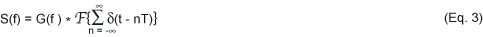Further, note that the asterisk in Equation 3 denotes convolution, not multiplication. Since we know the spectrum of the original signal, g(t), we need find only the Fourier transform of the train of impulses. To do so, we recognize that the train of impulses is a periodic function, and can therefore be represented by a Fourier series. Consequently, we write: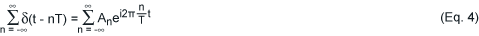Where the Fourier coefficients are: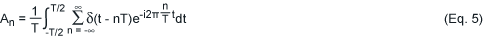The limits of integration for Equation 5 are specified only for one period. That is not a problem when dealing with the delta function. However, to make the above expressions more robust, note that substitutions can be made: The integral can be replaced with a Fourier integral from minus infinity to infinity, and the periodic train of delta functions can be replaced with a single delta function—the basis for the periodic signal. Thus, we can rewrite Equation 5 as: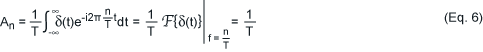The train of delta functions then assumes the following simplified expression, which is easily Fourier transformable: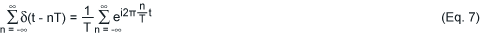Recognizing that a signal can be synthesized from its Fourier transform,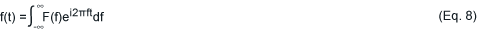And also that,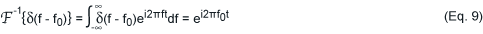We can finally write:Having obtained this result, we again focus our attention on the sampled baseband signal. We can now express its Fourier transform as follows:The convolution of two signals A(f) and B(f) is defined as:And we can express S(f) as: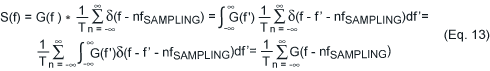Equation 13, commonly called the sampling theorem, is the result for which we have been working. It shows that sampling in the time domain at intervals of T seconds replicates the spectrum of our unsampled signal every 1/T cycles per second. Figure 2 shows our result in graphic form. That result, in turn, now allows us to answer the original question clearly and intuitively: How do we sample in a way that preserves the full information of the original signal?### The Effects of Aliasing

To preserve all information in the unsampled baseband signal, we must ensure that the spectrum "islands" do not overlap when replicating the spectrum. If they do (a phenomenon called aliasing), we can no longer extract the original signal from the samples. Aliasing allows higher frequencies to disguise themselves as lower frequencies, as can be seen in Figure 3.To avoid aliasing, you must preserve the following condition: 1/T ≥ 2α, or 1/T ≥ 2BW. This result can be expressed in terms of the sampling frequency: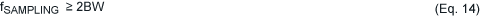Thus, the minimum sampling frequency necessary for sampling without aliasing is 2BW. This result is generally known as the Nyquist criterion.

Figure 3 depicts a sampled signal suffering from aliasing. Note that the high-frequency component, fH, appears at a much lower frequency. You can recover a signal from its sampled version by using a lowpass filter to isolate the original spectrum, and cutting (attenuating) everything else. Thus, extracting the signal with a lowpass filter of cutoff frequency α does not eliminate the aliased high frequency, but allows it to corrupt the signal of interest.

Recalling that aliasing can corrupt the signal of interest, consider a special class of band-limited signals known as bandpass signals. A bandpass signal is characterized by a bandwidth not bounded by zero at its lower end. To illustrate, the bandpass signal shown in Figure 4 has signal energy between the frequencies αL and αU, and its bandwidth is defined as αU - αL. Thus, the main difference between bandpass and baseband signals is in their definition of bandwidth: The bandwidth of a baseband signal equals its highest frequency, while the bandwidth of a bandpass signal is the difference between its upper- and lower-bound frequencies.From the discussion above, we know that by sampling this signal we replicate its spectrum at intervals of 1/T. Because that spectrum includes a substantial zero-amplitude band between zero and the signal's lower frequency bound, the actual signal bandwidth is smaller than αU. We can, therefore, use smaller shifts in the frequency domain, which allows a sampling frequency lower than that required for a signal whose spectrum occupies all frequencies from zero to αU. Assume, for example, a signal bandwidth of αU/2. To satisfy the Nyquist criterion our sampling frequency equals αU, producing the sampled-signal spectrum of Figure 5.You can see that this sampling produces no aliasing. We could, therefore, extract the original signal from the samples if we had a perfect bandpass filter. It is important to note in this example the difference between a baseband signal and a bandpass signal. For baseband signals, the bandwidth and hence the sampling frequency depend solely on the highest frequency present. For bandpass signals, bandwidth is usually smaller than the highest frequency.

These characteristics determine the method for recovering the sampled signal. Consider a baseband and a bandpass signal, each with the same value of maximum frequency. The bandpass signal permits a lower sampling frequency only if the recovery method includes a bandpass filter that isolates the original signal spectrum (the white rectangles in Figure 5). A lowpass filter used for baseband recovery cannot recover the original bandpass signal, because it includes the shaded areas shown in Figure 5. Thus, if you use a lowpass filter to recover the bandpass signal in Figure 5, you must sample at 2αU to avoid aliasing.

Thus, band-limited signals can be sampled and fully recovered only when observing the Nyquist criterion. For bandpass signals the Nyquist criterion will ensure no aliasing only when the recovery of the signal is done with a bandpass filter; otherwise a higher sampling frequency will be required. This knowledge is important when choosing the sampling frequency of any analog to digital converter.

One last consideration is our assumption of band-limited signals. Mathematically, a signal can never be truly band-limited. A law of Fourier transformations says that if a signal is finite in time, its spectrum extends to infinite frequency, and if its bandwidth is finite, its duration is infinite in time. Clearly we cannot have a time-domain signal of infinite duration, so we can never have a truly band-limited signal. Most practical signals, however, concentrate most of their energy in a definite portion of the spectrum. The analysis above is effective for such signals.

### Sampling Sinusoidal Signals

A very easy, convenient, and practical way of illustrating the disguising of higher frequencies as lower frequencies that are inherent in aliasing is by sampling sinusoidal signals. This is because pure sinusoidal signals have spectrums consisting only of spikes (delta functions) at the respective frequency; aliasing with pure tones is seen as the spike moving from one location to another. This other location is referred to as the image and is, in reality, the aliased signal.

The results presented below were taken with the MAX19541, 125Msps, 12-bit ADC. Figure 6 shows the spectrum at the converter's output for the input frequency fIN = 11.5284MHz. The data shows that the main spike occurs exactly at this frequency. There are a number of other spikes which are harmonics introduced by the nonlinearities of the converter, but they are irrelevant to our discussion. The sampling frequency, fSAMPLING = 125MHz, is more than twice the input frequency as required by the Nyquist criterion, and therefore no aliasing occurs.Next, consider what will happen to the location of the main spike if we increase the input frequency to fIN = 183.4856MHz. This input frequency is higher than fSAMPLING/2, and we expect aliasing to occur. The resulting spectrum given in Figure 7 shows that the main spike is now located at 58.48MHz, and this is the aliased signal. In other words, an image has appeared at 58.48MHz when, in fact, our input signal did not contain this frequency. Note that in both Figures 6 and 7 we plotted the spectrum only up to the Nyquist frequency. This is because the spectrum is periodic and this portion contains all the essential information.